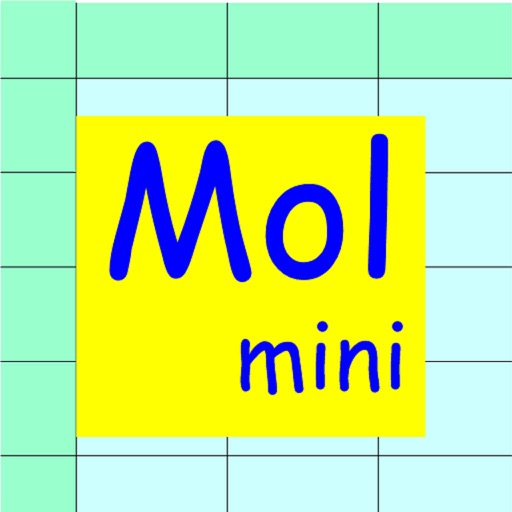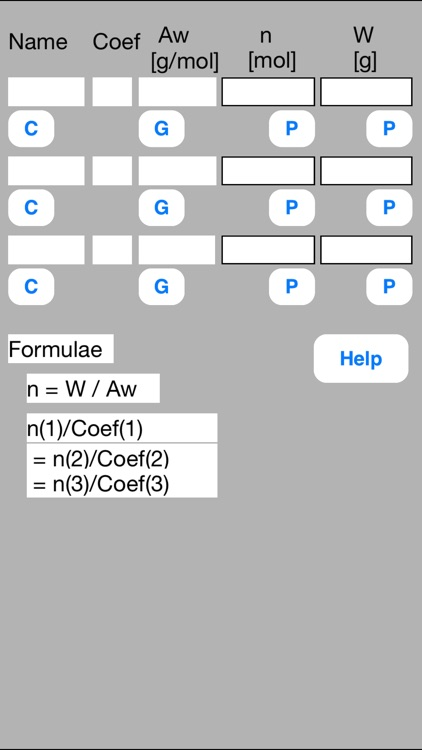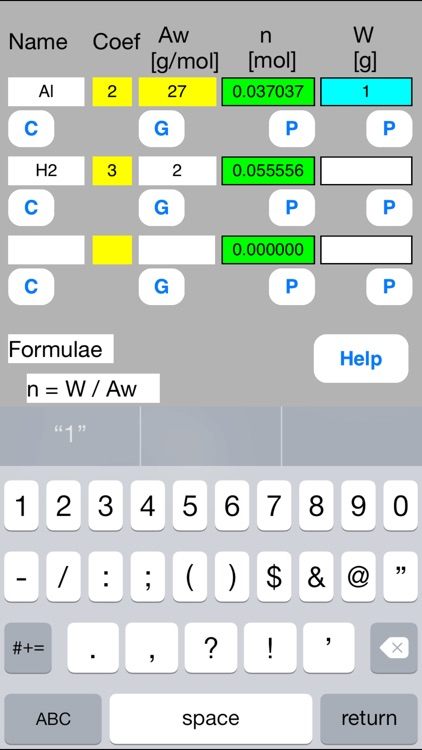## Mol calculator mini is a calculation sheet that solves chemical mol calculation problems# Mol Calculator mini

by Mineo YamauchiMol calculator mini is a calculation sheet that solves chemical mol calculation problems. This Mol calculator mini is a subset of iPad Mol Calculator software.### App Details

Version
1.07
Rating
NA
Size
0Mb
Genre
Utilities Education
Last updated
November 23, 2016
Release date
August 15, 2012

### App Screenshots### App Store Description

Mol calculator mini is a calculation sheet that solves chemical mol calculation problems. This Mol calculator mini is a subset of iPad Mol Calculator software.
This software solves the problem not as a black box but as a white box. You need to think the process of solving a problem. This makes your knowledge refresh. The black box solution is convenient, however it has a risk of losing your knowledge. This software has few such risks.

A typical problem is as follows.

(Example)
The hydrogen gas used for a fuel cell is generated from aluminum powder particles and water. How much of hydrogen gas can be generated from 1 g of aluminum?

Mol calculator mini solves the problem in the following three steps.

//Step 0: Preparation//
Prepare chemical equations and atomic (molecular) weights. They are basics for the calculation.
They are as follows.
2Al + 6H2O -> 2Al(OH)3 + 3H2
Atomic weight of Al = 27 [g/mol]
Molecular weight of H2 = 2 [g/mol]

//Step 1: Definition//
Enter known data into the Mol mini sheet.
Rows correspond to substances. Columns correspond to the attributes of substance.
First row
Name = Al, Coef = 2, Aw = 27, W = 1
Second row
Name = H2, Coef = 3, Aw = 2

//Step 2: Calculation//
Attribute data are not independent but mutually related by formulae.
Calculation is a conversion from unknown data to known data using the formulae.
The formulae are embedded in P and G buttons.
Touching out those buttons executes the formulae and changes unknown data to known data.
Touch out buttons as follows.
Touch out P_W button of the first row. Mol data are changed to known data.
Touch out P_Mol button of the second row. W data are changed to known data.
It turns out that the mol and gram of hydrogen gas are 0.0555 mol and 0.1111 g respectively.

[Data label]
Name: Substance name (option).
Coef: Substance coefficient of chemical equation.
This defines quantity ratio among substances.
When a chemical equation is aX + bY -> cZ, then a, b, and c are coefficients.
When the Coef text of the data field is not numeric, it is interpreted as zero value.
Aw [g/mol]: Atomic or molecular weight of substance
n [mol]: Mol quantity of substance.
W [g]: Weight of substance.

[Buttons and data]
//P button and P data group//
P (put) button inputs data to which the P button is assigned.
P data group is a group of data to which the P buttons are assigned. The P data group has a special character. When one P data is input, all other data of the P data group are updated by formulae. Ｗhen the P button is touched out, Mol data of other rows are also updated by use of Coef data.
When the data field is blank, it is interpreted as illegal data.
When the data field is illegal character, it is interpreted as zero value.
Number of input data: number of output data = 1: N, where N > 1.

//G button//
G (get) button gets data from other data using formulae.
When the data field is blank, it is interpreted as illegal data.
When the data field is illegal character, it is interpreted as zero value.
Number of input data: number of output data = N: 1, where N > 1.

//C button//
C (Clear) button clears all row data.

[Color change]
Touching out a button executes calculation and generates output data from the input and the referenced data. Unknown data are changed to known data.
Color change shows what data are input, output, or referenced data in this button execution.
Input data:Blue color
Referenced data:Yellow color
Output data:Green color
Uncalculated data:Red color
Referenced data is an input data that is referenced at the P button execution.
Uncalculated data is an output data that is not normally calculated. This uncalculated data is not output. The former data is remains unchanged in this data field.

[Formulae]
Embedded formulae are as follows.
n = W / Aw
n(1)/Coef(1) = n(2)/Coef(2) = n(3)/Coef(3)

Disclaimer:
AppAdvice does not own this application and only provides images and links contained in the iTunes Search API, to help our users find the best apps to download. If you are the developer of this app and would like your information removed, please send a request to [email protected] and your information will be removed.